Скачать презентацию Chapter 21 Savings Models Lesson Plan n Arithmetic

e9ef90f63fa8b97def97cb747821a1f1.ppt

• Количество слайдов: 17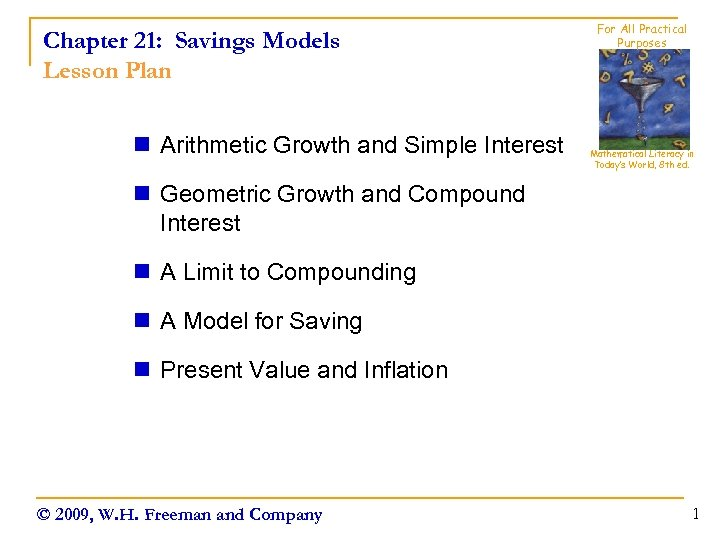Chapter 21: Savings Models Lesson Plan n Arithmetic Growth and Simple Interest For All Practical Purposes Mathematical Literacy in Today’s World, 8 th ed. n Geometric Growth and Compound Interest n A Limit to Compounding n A Model for Saving n Present Value and Inflation © 2009, W. H. Freeman and Company 1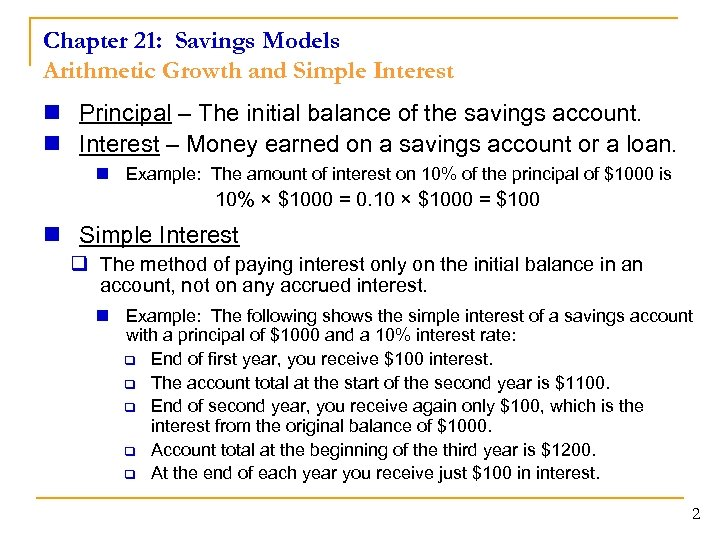Chapter 21: Savings Models Arithmetic Growth and Simple Interest n Principal – The initial balance of the savings account. n Interest – Money earned on a savings account or a loan. n Example: The amount of interest on 10% of the principal of \$1000 is 10% × \$1000 = 0. 10 × \$1000 = \$100 n Simple Interest q The method of paying interest only on the initial balance in an account, not on any accrued interest. n Example: The following shows the simple interest of a savings account with a principal of \$1000 and a 10% interest rate: q End of first year, you receive \$100 interest. q The account total at the start of the second year is \$1100. q End of second year, you receive again only \$100, which is the interest from the original balance of \$1000. q Account total at the beginning of the third year is \$1200. q At the end of each year you receive just \$100 in interest. 2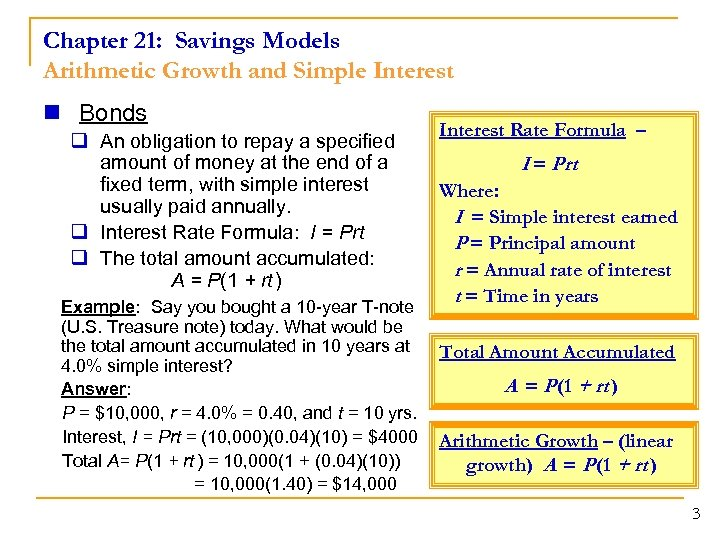Chapter 21: Savings Models Arithmetic Growth and Simple Interest n Bonds q An obligation to repay a specified amount of money at the end of a fixed term, with simple interest usually paid annually. q Interest Rate Formula: I = Prt q The total amount accumulated: A = P(1 + rt ) Example: Say you bought a 10 -year T-note (U. S. Treasure note) today. What would be the total amount accumulated in 10 years at 4. 0% simple interest? Answer: P = \$10, 000, r = 4. 0% = 0. 40, and t = 10 yrs. Interest, I = Prt = (10, 000)(0. 04)(10) = \$4000 Total A= P(1 + rt ) = 10, 000(1 + (0. 04)(10)) = 10, 000(1. 40) = \$14, 000 Interest Rate Formula – I = Prt Where: I = Simple interest earned P = Principal amount r = Annual rate of interest t = Time in years Total Amount Accumulated A = P (1 + rt ) Arithmetic Growth – (linear growth) A = P (1 + rt ) 3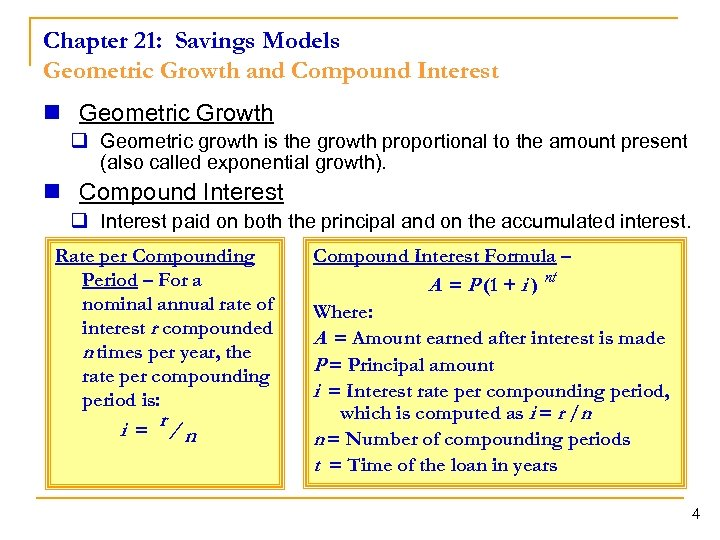Chapter 21: Savings Models Geometric Growth and Compound Interest n Geometric Growth q Geometric growth is the growth proportional to the amount present (also called exponential growth). n Compound Interest q Interest paid on both the principal and on the accumulated interest. Rate per Compounding Period – For a nominal annual rate of interest r compounded n times per year, the rate per compounding period is: i = r/ n Compound Interest Formula – A = P (1 + i ) nt Where: A = Amount earned after interest is made P = Principal amount i = Interest rate per compounding period, which is computed as i = r /n n = Number of compounding periods t = Time of the loan in years 4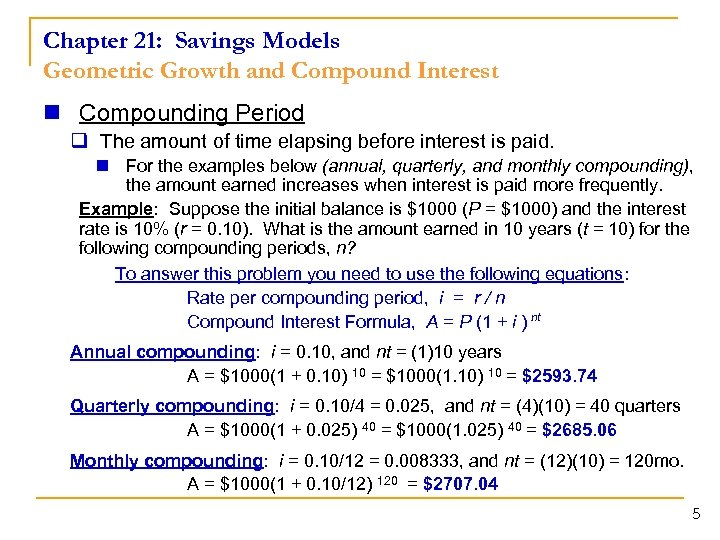Chapter 21: Savings Models Geometric Growth and Compound Interest n Compounding Period q The amount of time elapsing before interest is paid. n For the examples below (annual, quarterly, and monthly compounding), the amount earned increases when interest is paid more frequently. Example: Suppose the initial balance is \$1000 (P = \$1000) and the interest rate is 10% (r = 0. 10). What is the amount earned in 10 years (t = 10) for the following compounding periods, n? To answer this problem you need to use the following equations: Rate per compounding period, i = r / n Compound Interest Formula, A = P (1 + i ) nt Annual compounding: i = 0. 10, and nt = (1)10 years A = \$1000(1 + 0. 10) 10 = \$1000(1. 10) 10 = \$2593. 74 Quarterly compounding: i = 0. 10/4 = 0. 025, and nt = (4)(10) = 40 quarters A = \$1000(1 + 0. 025) 40 = \$1000(1. 025) 40 = \$2685. 06 Monthly compounding: i = 0. 10/12 = 0. 008333, and nt = (12)(10) = 120 mo. A = \$1000(1 + 0. 10/12) 120 = \$2707. 04 5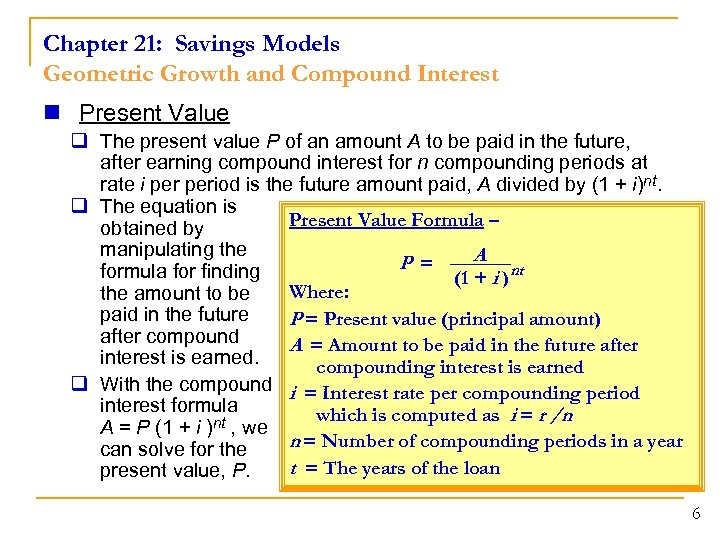Chapter 21: Savings Models Geometric Growth and Compound Interest n Present Value q The present value P of an amount A to be paid in the future, after earning compound interest for n compounding periods at rate i period is the future amount paid, A divided by (1 + i)nt. q The equation is Present Value Formula – obtained by manipulating the A P = formula for finding (1 + i )nt Where: the amount to be paid in the future P = Present value (principal amount) after compound A = Amount to be paid in the future after interest is earned. compounding interest is earned q With the compound i = Interest rate per compounding period interest formula which is computed as i = r /n A = P (1 + i )nt , we n = Number of compounding periods in a year can solve for the t = The years of the loan present value, P. 6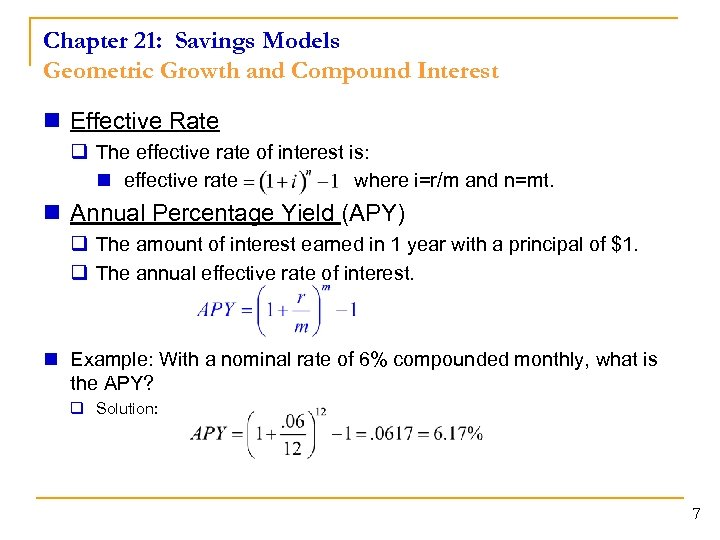Chapter 21: Savings Models Geometric Growth and Compound Interest n Effective Rate q The effective rate of interest is: n effective rate where i=r/m and n=mt. n Annual Percentage Yield (APY) q The amount of interest earned in 1 year with a principal of \$1. q The annual effective rate of interest. n Example: With a nominal rate of 6% compounded monthly, what is the APY? q Solution: 7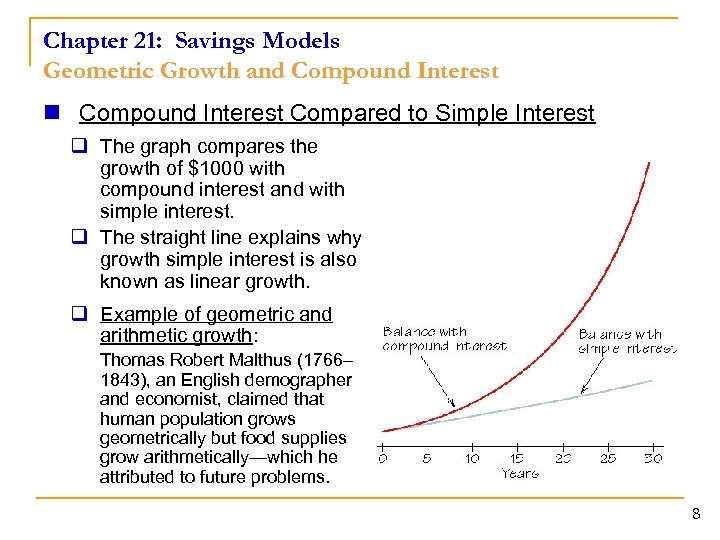Chapter 21: Savings Models Geometric Growth and Compound Interest n Compound Interest Compared to Simple Interest q The graph compares the growth of \$1000 with compound interest and with simple interest. q The straight line explains why growth simple interest is also known as linear growth. q Example of geometric and arithmetic growth: Thomas Robert Malthus (1766– 1843), an English demographer and economist, claimed that human population grows geometrically but food supplies grow arithmetically—which he attributed to future problems. 8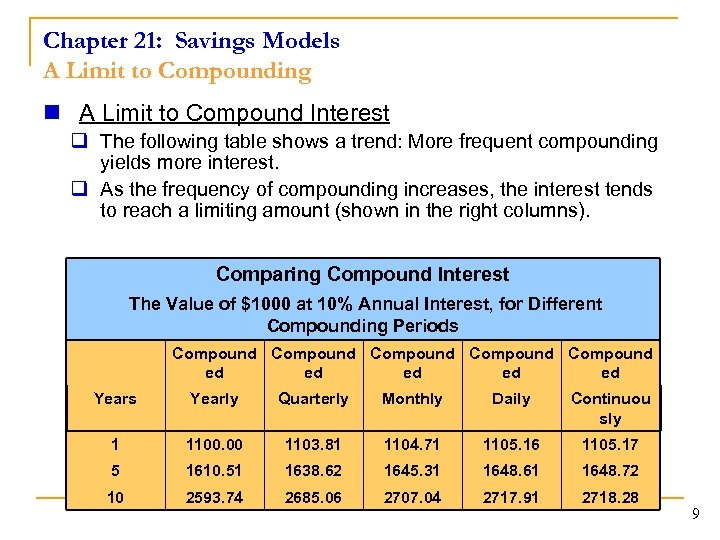Chapter 21: Savings Models A Limit to Compounding n A Limit to Compound Interest q The following table shows a trend: More frequent compounding yields more interest. q As the frequency of compounding increases, the interest tends to reach a limiting amount (shown in the right columns). Comparing Compound Interest The Value of \$1000 at 10% Annual Interest, for Different Compounding Periods Compound Compound ed ed ed Years Yearly Quarterly Monthly Daily Continuou sly 1 1100. 00 1103. 81 1104. 71 1105. 16 1105. 17 5 1610. 51 1638. 62 1645. 31 1648. 61 1648. 72 10 2593. 74 2685. 06 2707. 04 2717. 91 2718. 28 9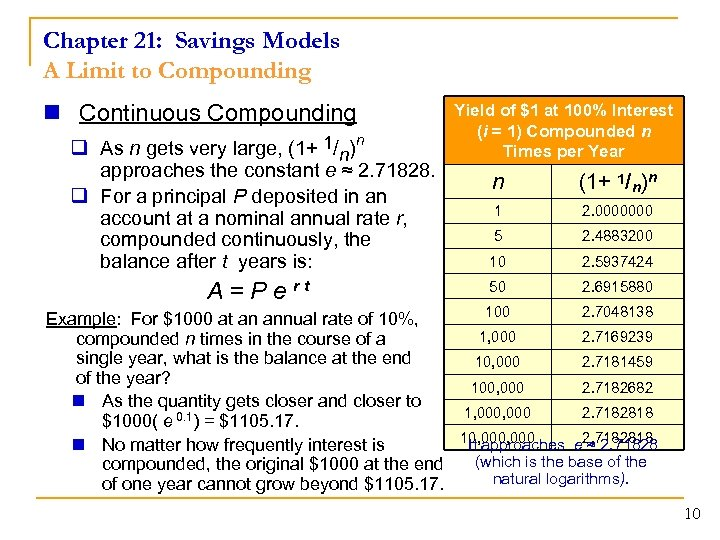Chapter 21: Savings Models A Limit to Compounding n Continuous Compounding q As n gets very large, (1+ 1/n)n approaches the constant e ≈ 2. 71828. q For a principal P deposited in an account at a nominal annual rate r, compounded continuously, the balance after t years is: A = P e rt Yield of \$1 at 100% Interest (i = 1) Compounded n Times per Year n (1+ 1/n)n 1 2. 0000000 5 2. 4883200 10 2. 5937424 50 2. 6915880 100 2. 7048138 Example: For \$1000 at an annual rate of 10%, 1, 000 2. 7169239 compounded n times in the course of a single year, what is the balance at the end 10, 000 2. 7181459 of the year? 100, 000 2. 7182682 n As the quantity gets closer and closer to 1, 000 2. 7182818 \$1000( e 0. 1) = \$1105. 17. 10, 000 It approaches e 2. 7182818 ≈ 2. 71828 n No matter how frequently interest is (which is the base of the compounded, the original \$1000 at the end natural logarithms). of one year cannot grow beyond \$1105. 17. 10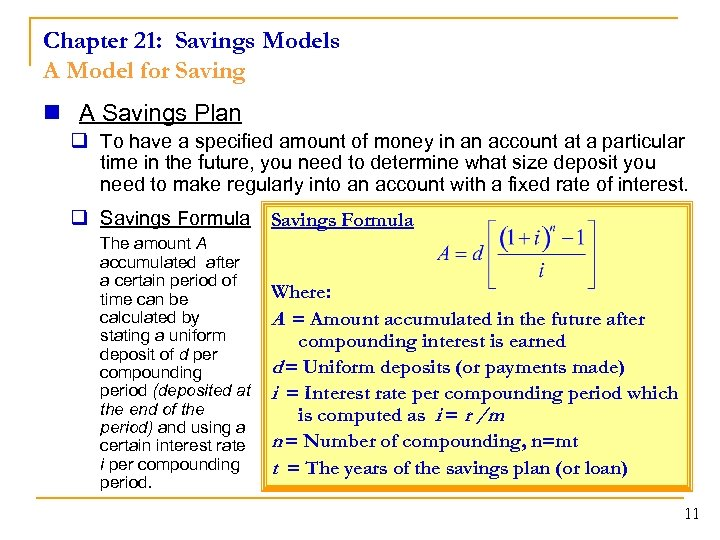Chapter 21: Savings Models A Model for Saving n A Savings Plan q To have a specified amount of money in an account at a particular time in the future, you need to determine what size deposit you need to make regularly into an account with a fixed rate of interest. q Savings Formula The amount A accumulated after a certain period of time can be calculated by stating a uniform deposit of d per compounding period (deposited at the end of the period) and using a certain interest rate i per compounding period. Savings Formula Where: A = Amount accumulated in the future after compounding interest is earned d = Uniform deposits (or payments made) i = Interest rate per compounding period which is computed as i = r /m n = Number of compounding, n=mt t = The years of the savings plan (or loan) 11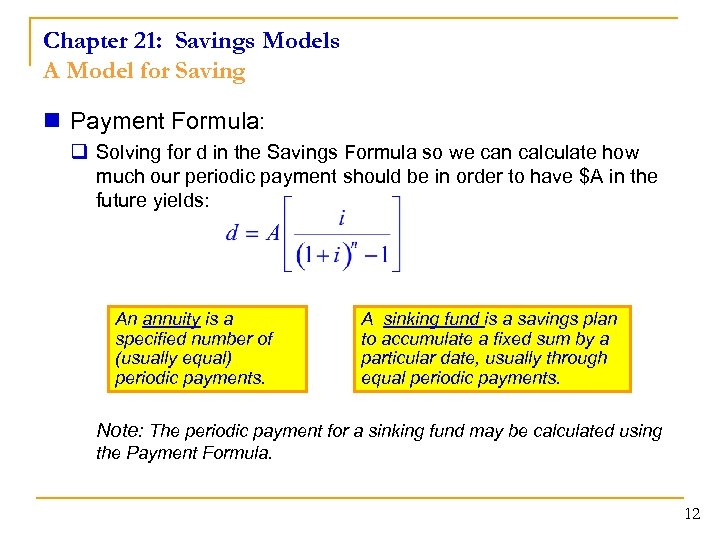Chapter 21: Savings Models A Model for Saving n Payment Formula: q Solving for d in the Savings Formula so we can calculate how much our periodic payment should be in order to have \$A in the future yields: An annuity is a specified number of (usually equal) periodic payments. A sinking fund is a savings plan to accumulate a fixed sum by a particular date, usually through equal periodic payments. Note: The periodic payment for a sinking fund may be calculated using the Payment Formula. 12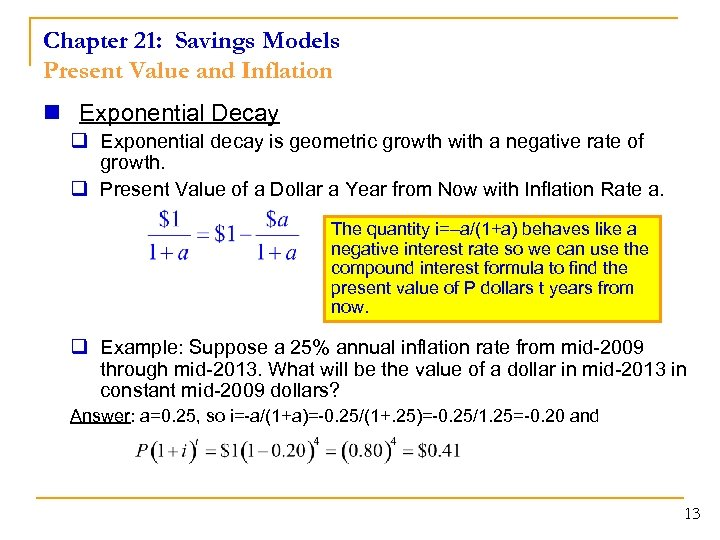Chapter 21: Savings Models Present Value and Inflation n Exponential Decay q Exponential decay is geometric growth with a negative rate of growth. q Present Value of a Dollar a Year from Now with Inflation Rate a. The quantity i=–a/(1+a) behaves like a negative interest rate so we can use the compound interest formula to find the present value of P dollars t years from now. q Example: Suppose a 25% annual inflation rate from mid-2009 through mid-2013. What will be the value of a dollar in mid-2013 in constant mid-2009 dollars? Answer: a=0. 25, so i=-a/(1+a)=-0. 25/(1+. 25)=-0. 25/1. 25=-0. 20 and 13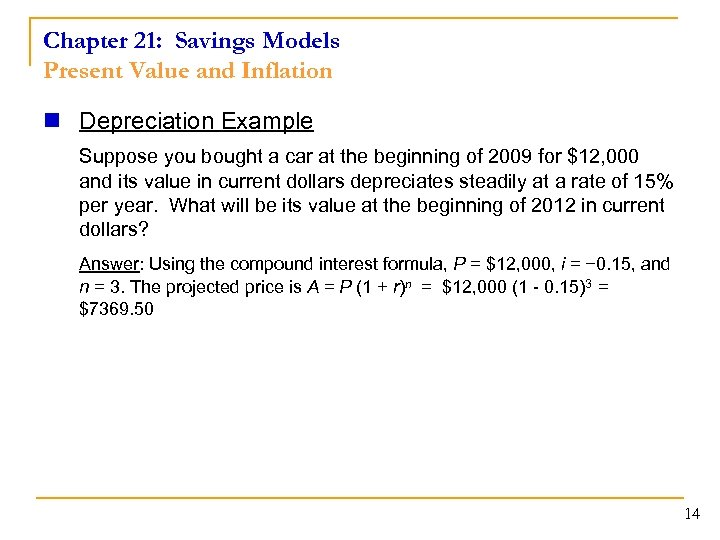Chapter 21: Savings Models Present Value and Inflation n Depreciation Example Suppose you bought a car at the beginning of 2009 for \$12, 000 and its value in current dollars depreciates steadily at a rate of 15% per year. What will be its value at the beginning of 2012 in current dollars? Answer: Using the compound interest formula, P = \$12, 000, i = − 0. 15, and n = 3. The projected price is A = P (1 + r)n = \$12, 000 (1 - 0. 15)3 = \$7369. 50 14Chapter 21: Savings Models Present Value and Inflation n Consumer Price Index q The official measure of inflation is the Consumer Price Index (CPI), prepared by the Bureau of Labor Statistics. n This index represents all urban consumers (CPI-U) and covers about 80% of the U. S. population. n This is the index of inflation that is referred to on television news broadcasts, in newspapers, and magazine articles. n Each month, the Bureau of Labor Statistics determines the average cost of a “market basket” of goods, including food, housing, transportation, clothing, and other items. n The base period used to construct the CPI-U is from 1982– 1984 and is set to 100. CPI for other year cost of market basket in = other year basket in base year 100 cost of market n From this proportion calculation, you can also compute the cost of an = item in dollars for one year to what it would cost in dollars in a different year. cost in year A CPI for year A 15 cost in year B CPI for year B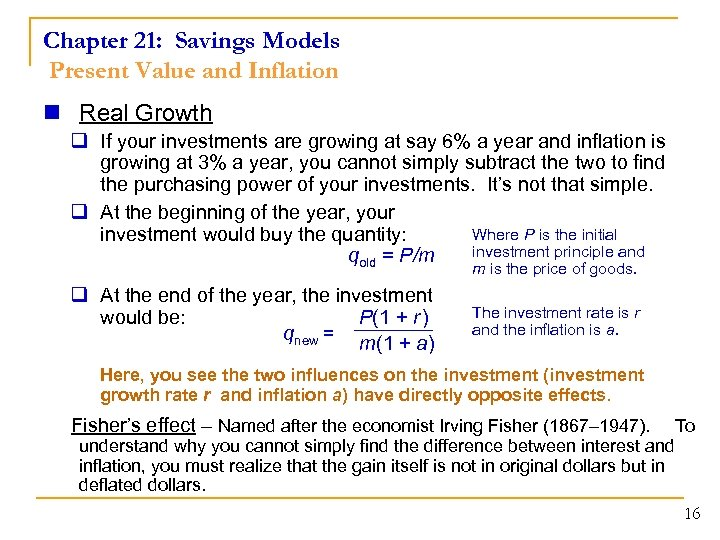Chapter 21: Savings Models Present Value and Inflation n Real Growth q If your investments are growing at say 6% a year and inflation is growing at 3% a year, you cannot simply subtract the two to find the purchasing power of your investments. It’s not that simple. q At the beginning of the year, your Where P is the initial investment would buy the quantity: investment principle and qold = P/m m is the price of goods. q At the end of the year, the investment would be: P(1 + r ) qnew = m(1 + a) The investment rate is r and the inflation is a. Here, you see the two influences on the investment (investment growth rate r and inflation a) have directly opposite effects. Fisher’s effect – Named after the economist Irving Fisher (1867– 1947). To understand why you cannot simply find the difference between interest and inflation, you must realize that the gain itself is not in original dollars but in deflated dollars. 16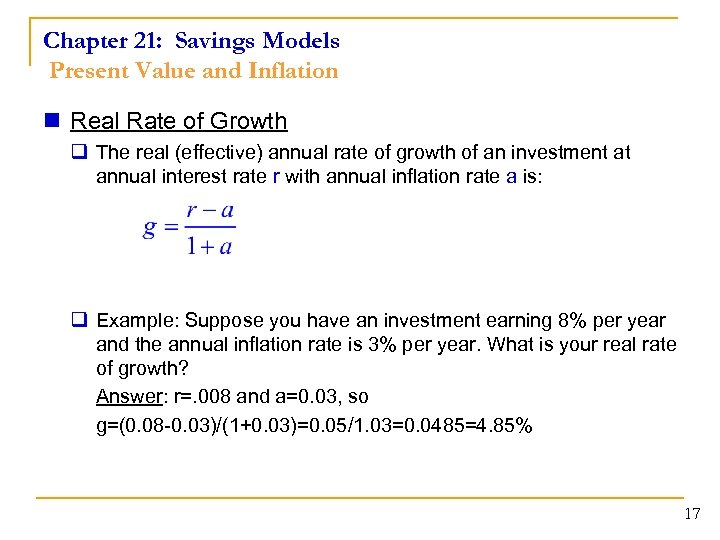Chapter 21: Savings Models Present Value and Inflation n Real Rate of Growth q The real (effective) annual rate of growth of an investment at annual interest rate r with annual inflation rate a is: q Example: Suppose you have an investment earning 8% per year and the annual inflation rate is 3% per year. What is your real rate of growth? Answer: r=. 008 and a=0. 03, so g=(0. 08 -0. 03)/(1+0. 03)=0. 05/1. 03=0. 0485=4. 85% 17